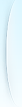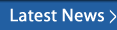Call: Anusha @ 1 (877) 864-8462 Loading input.gsc-input { border-color:#5B9000; } input.gsc-search-button { border-color:#000000; color:#FFFFFF; background-color:#5B9000; }HomeDB/UNIXUNIX/LINUXAWK COMMAND IN UNIX
DB/UNIX
AWK COMMAND IN UNIX
AWK COMMAND IN UNIX

# Awk command

awk -- it allows the user to manipulate files that are structured as columns of data and strings, JUST SEE THE FIRST EXAMPLE U WILL GET IDEA

Example 1:
Assume you want to process a file called file 'dimensions' that has the following content:

12 8
15 24
9 12

Assume you want to generate a file 'area' that has the same content as the file 'dimensions', but has one more column that contains the product of the two numbers on each line:

12 8 96
15 24 360
9 12 108

The following command would accomplish that:

awk '{print \$0, \$1*\$2}' dimenions > area

awk -- it allows the user to manipulate files that are structured as columns of data and strings. (--continued after the ads...)

Once you understand the basics of awk you will find that it is surprisingly useful. You can use it to automate things in ways you have never thought about. It can be used for data processing and for automating the application of Linux / Unix commands. It also has many spreadsheet-type functionalities.

There are two ways to run awk:

1. A simple awk command can be run from the command line.
2. More complex tasks should be written as awk programs ("scripts") to a file. Examples of each are provided below. A simple awk command is of the form

% awk 'pattern {action}' input-file > output-file

meaning: take each line of the input file; if the line contains the pattern apply the action to the line and write the resulting line to the output-file.

If the pattern is omitted, the action is applied to all lines:

% awk '{action}' input-file > output-file

By default, awk works on files that have columns of numbers or strings that are separated by white space (tabs or spaces), but the -F option can be used if the columns are separated by another character. awk refers to the first column as \$1, the second column as \$2, etc. The whole line referred to as \$0.

Example 1:

Assume you want to process a file called file 'dimensions' that has the following content:

12 8
15 24
9 12

Assume you want to generate a file 'area' that has the same content as the file 'dimensions', but has one more column that contains the product of the two numbers on each line:

12 8 96
15 24 360
9 12 108

The following command would accomplish that:

awk '{print \$0, \$1*\$2}' dimenions > area

The term {print \$0, \$1*\$2} means: first print the whole line (\$0), then print the product of the number in column 1 (\$1) and the number in column 2 (\$2).

Example 2:

If you want the output file to contain only those lines on which the first number is less than the second number, you would use the following command:

awk '\$1 < \$2 {print \$0, \$1*\$2}' dimenions > area2

The contents of file area2 would then be:

15 24 360
9 12 108

Example 3:

The following command does exactly the same as the command in Example 2, but it illustrates how awk can be combined with other Unix commands:

cat dimenions | awk '\$1 < \$2 {print \$0, \$1/\$2}' > area2

Used by itself, the command 'cat dimenions' simply prints the contents of file 'dimenions' to the sceen. However, if a command is followed by a '|' (called "pipe"), the contents goes as input to command to the right of the '|'.

Example 4:

Assume you have hundreds of files you want to move into a new directory and rename them by appending a .new to the filenames. Assume all the files have names that start with "data", they need to be moved to ../newdata, and need to have a '.new' appended to the name. Use the following command to accomplish this:

ls data* | awk '{print "mv "\$0" ../newdata/"\$0".new"}' | csh

ls data* lists the filenames. This output list is then "piped" into awk. Since there is no pattern specified, awk proceeds to print something for each line. For example, if the first two lines from 'ls data*' produced data1 and data2, respectively, then awk would print:

mv data1 ../newdata/data1.new
mv data2 ../newdata/data2.new

These are Unix commands that are executed by piping them into the "csh" command ("csh" is an operating system shell).

More complex awk scripts need to be run from a file. The syntax for such cases is:

cat file-1 | awk -f script-1.awk > file-2

where file-1 is the input file, file-2 is the output file, and script-1.awk is a file containing awk commands. awk scripts that contain more than one line need to be run from files. The following example is an awk-script that would saved in a file (e.g, script-1.awk) and executed by the above command.

Example 5:

The following awk-script prints frequency a histogram of the first column of the input file (assumed to contain numbers):

(\$1 > 0.1) && (\$1 <= 0.2) {num_b = num_b+1}
(\$1 > 0.2) && (\$1 <= 0.3) {num_c = num_c+1}
(\$1 > 0.3) && (\$1 <= 0.4) {num_d = num_d+1}
(\$1 > 0.4) && (\$1 <= 0.5) {num_e = num_e+1}
(\$1 > 0.5) && (\$1 <= 0.6) {num_f = num_f+1}
(\$1 > 0.6) && (\$1 <= 0.7) {num_g = num_g+1}
(\$1 > 0.7) {num_h = num_h+1}
END {print num_a, num_b, num_c, num_d, num_e, num_f, num_g, num_h}

Note that each line contains an instruction of the form pattern {action}. All instructions are executed sequentially. The "pattern" 'END' is satisfied when the end of the input file is reached.

Other useful awk-variables are:
· NF: number of columns;
· NR: the current line that awk is working on;
· BEGIN: satisfied before anything is read;
· length: number of characters in a line or a string;

awk also provides looping capability, a search (/) command, a substring command, and formatted printing. It provides the logical operators || (or) and && (and) that can be used in specifying patterns. You can define variables and assign values to them.Krishna Training Courses Offered AWS Certified Solutions A Quality Assurance Course © 2005 - 2020 Krishna Training. Designed & Developed By BitraNet